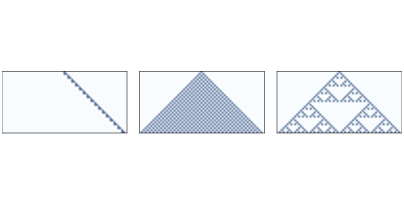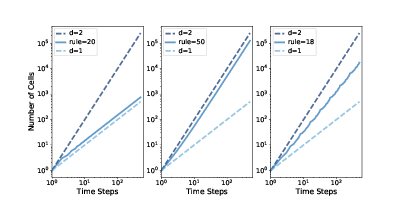# 9.6. Fractals¶

For simple geometric objects, dimension is defined in terms of scaling behavior. For example, if the side of a square has length $$l$$, its area is $$l^2$$. The exponent, 2, indicates that a square is two-dimensional. Similarly, if the side of a cube has length $$l$$, its volume is $$l^3$$, which indicates that a cube is three-dimensional.

More generally, we can estimate the dimension of an object by measuring some kind of size (like area or volume) as a function of some kind of linear measure (like the length of a side).

As an example, We’ll estimate the dimension of a 1-D cellular automaton by measuring its area (total number of “on” cells) as a function of the number of rows.Figure 9.6: One-dimensional CAs with rules 20, 50, and 18, after 32 time steps.¶

Figure 9.6 shows three 1-D CAs like the ones we saw in Section 7.2. Rule 20 (left) generates a set of cells that seems like a line, so we expect it to be one-dimensional. Rule 50 (center) produces something like a triangle, so we expect it to be 2-D. Rule 18 (right) also produces something like a triangle, but the density is not uniform, so its scaling behavior is not obvious.

We’ll estimate the dimension of these CAs with the following function, which counts the number of on cells after each time step. It returns a list of tuples, where each tuple contains i, $$i^2$$, and the total number of cells.

def count_cells(rule, n=500):
ca = Cell1D(rule, n)
ca.start_single()

res = []
for i in range(1, n):
cells = np.sum(ca.array)
res.append((i, i**2, cells))
ca.step()

return resFigure 9.7: Number of “on” cells versus number of time steps for rules 20, 50, and 18.¶

Figure 9.7 shows the results plotted on a log-log scale.

In each figure, the top dashed line shows $$y = i^2$$. Taking the log of both sides, we have $$log y = 2 log i$$. Since the figure is on a log-log scale, the slope of this line is 2.

Similarly, the bottom dashed line shows $$y = i$$. On a log-log scale, the slope of this line is 1.

Rule 20 (left) produces 3 cells every 2 time steps, so the total number of cells after i steps is $$y = 1.5 i$$. Taking the log of both sides, we have $$log y = log 1.5 + log i$$, so on a log-log scale, we expect a line with slope 1. In fact, the estimated slope of the line is 1.01.

Rule 50 (center) produces $$i+1$$ new cells during the ith time step, so the total number of cells after i steps is $$y = i^2 + i$$. If we ignore the second term and take the log of both sides, we have $$log y ∼ 2 log i$$, so as i gets large, we expect to see a line with slope 2. In fact, the estimated slope is 1.97.

Finally, for Rule 18 (right), the estimated slope is about 1.57, which is clearly not 1, 2, or any other integer. This suggests that the pattern generated by Rule 18 has a “fractional dimension”; that is, it is a fractal.

This way of estimating a fractal dimension is called box-counting.